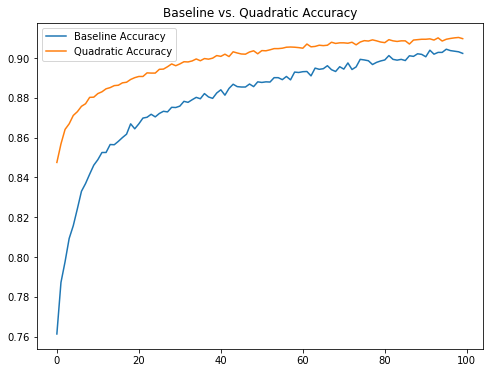# A Simple Quadratic Convolutional Layer in Tensorflow

Generally in computer vision research and applications the greatest successes have come from neural networks composed of various convolutional layers. More specifically, they are composed of linear convolutional layers.

As a refresher, in order to construct a linear convolutional layer applied to an input image $X$ in which the convolutional layer has kernel of size $k \times k$, we first decompose $X$ as a sequence of $k \times k$ patches. Then, we vectorize each patch, i.e., we reshape the patch as a vector of length $k^2$. Lastly, given each vectorized patch $x$, we compute a result given by

\begin{equation*} y = w^T x + b, \end{equation*}

where $w$ is a vector of length $k^2$, the kernel, and $b$ is the bias term. For a beautiful, lucid description of linear convolutions I recommend Christopher Olah's post.

Of course, in the description above I have intentionally ignored some very important details, such as what to do about patches on the boarder of the input (same or valid convolutions), dealing with stride length, etc? What is import to the discussion here, however, is the type of function that is applied to each patch.

The reason I have been stressing the 'linear' in linear convolutions is that the function applied to each patch is a typical linear function. In principle we need not constrain ourselves to linear functions, however, there are good reasons to do so. For one, linear functions are very easy to compute. In fact, many of the recent advances in machine learning can be attributed to our ability to find increasingly more effective ways to perform linear operations (gpus and the like). Another reason is that computing the gradient of a linear function is also very easy, making things like stochastic gradient descent possible and effective when used to optimized linear convolutional networks.

There are also reasons to try other functions besides linear. In a recent Arxiv paper, some authors tried what they called Volterra convolutional layers, or more generally quadratic layers. The idea here is that for each patch $x$ in the input image, we compute the value

\begin{equation*} x^T Q x + w^T x + b, \end{equation*}

where $w$ and $b$ are the same as those appearing in a linear convolution and $Q$ is a $k^2 \times k^2$ matrix which contributes a quadratic non-linearity.

It should be noted that this isn't the first time that a quadratic convolutional layer has been proposed in the neural computing literature. For example, some researchers proposed a similar extension however this was done a little while ago when computational facilities were not quite as good as they are now. In addition, the reason for trying a quadratic nonlinearity comes from research into the modelling of the eye and how it responds to various stimulus.

The researchers involved in the Volterra convolutions paper made their code available although for my purposes it was not terribly helpful. Mostly because it was written in Lua and CUDA code implementing the convolution itself. In order to play around with this type of filter I wrote a crude implementation in Tensorflow. Note that there is a slight difference in my implementation compared to the original author's. I do not take into account the symmetric nature of the quadratic form used and so in effect perform some redundant computations. This shouldn't affect the performance of the filter in terms of accuracy and applicability however.

def volterra_conv(inputs, W, input_dim, kernel_size, padding='SAME'):
input_patches = tf.extract_image_patches(inputs,
ksizes=[1, kernel_size, kernel_size, 1],
strides=[1, 1, 1, 1],
rates=[1, 1, 1, 1],
batch, out_row, out_col, sizes = input_patches.get_shape().as_list()
input_patches = tf.reshape(input_patches,
[-1, out_row, out_col, kernel_size*kernel_size, input_dim])
V = tf.einsum('abcid,abcjd,dijo->abcdo', input_patches, input_patches, W)
return tf.reduce_sum(V, 3)

def volterra_layer(inputs,
filters=32,
kernel_size=[3, 3],
activation=tf.nn.relu):
input_dim = inputs.get_shape().as_list()[-1]

W1 = tf.Variable(tf.truncated_normal([input_dim, kernel_size**2, kernel_size**2, filters]))
W2 = tf.Variable(tf.truncated_normal([kernel_size, kernel_size, input_dim, filters]))
b  = tf.Variable(tf.constant(0.1, shape=[filters]))Contents © 2017 Sean Carrell - Powered by Nikola